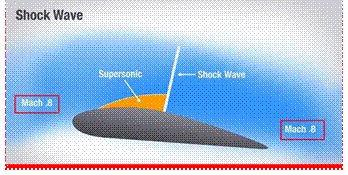#The ratio of velocity of fluid, in an undisturbed stream, to the velocity of sound wave, is known as Mach number.
It gives us an important information about the type of flow. In general, the flow of a fluid is divided into the following four types depending upon the Mach number.(a) When the Mach number is less than unity, the flow is called a sub-sonic flow.
(b) When the Mach number is equal to unity, the flow is called a sonic flow.
(c) When the Mach number is between 1 and 5, the flow is called a supersonic flow.
(d) When the Mach number is more than 5, the flow is called hypersonic flow.